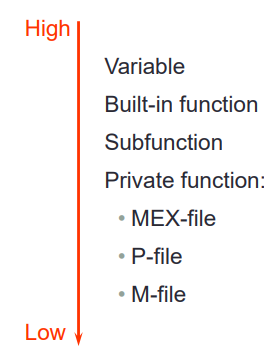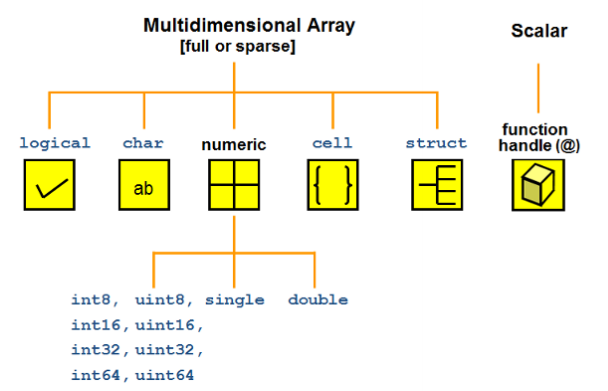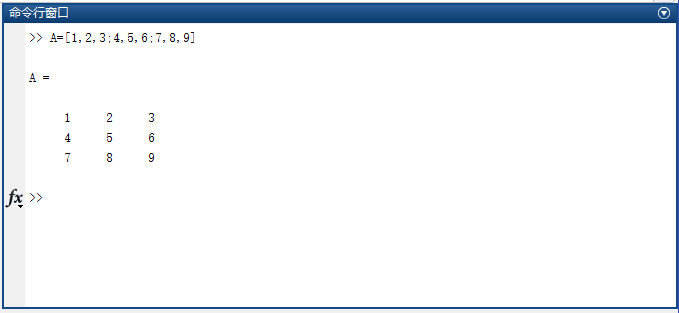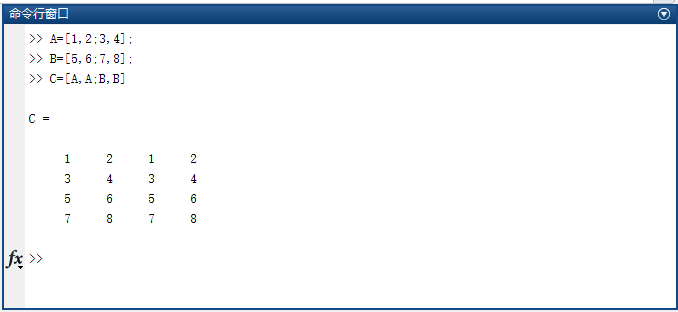• ## MATLAB01:基本的数学运算与矩阵运算

万次阅读 多人点赞 2019-11-12 17:22:52
MATLAB01:基本的数学运算与矩阵运算MATLAB基本语法变量变量名保留变量不适合做变量名变量不应当覆盖内置函数MATLAB的调用优先级变量类型数字型变量的显示格式MATLAB命令行使用MATLAB进行数字运算使用MATLAB计算数学...
pdf版本笔记的下载地址: MATLAB01_基本的数学运算与矩阵运算（访问密码：3834）
MATLAB01:基本的数学运算与矩阵运算MATLAB基本语法变量变量名保留变量不适合做变量名变量名不应当覆盖内置函数名变量类型数字型变量的显示格式MATLAB命令行使用MATLAB进行数字运算使用MATLAB计算数学表达式MATLAB内置的数学函数使用MATLAB进行矩阵运算定义矩阵向终端输入矩阵使用冒号运算符创建向量定义特殊矩阵矩阵的索引矩阵的操作操作矩阵的运算符操作矩阵的函数
学习一门技术最好的方式就是阅读官方文档,可以查看MATLAB官方文档
MATLAB基本语法
变量

MATLAB中的变量不需要声明.
使用=为变量赋值

变量名

与大多数编程语言相同,MATLAB中的变量名是大小写敏感的.
变量名只能由[0~9,a~z,A~z,_]组成,且变量名不能以数字开头.

保留变量不适合做变量名
MATLAB中有一些变量有其具体意义,不适合用作变量名.

变量
意义

ans
上一句的运算的结果

i和j
复数算子

Inf
无穷$\infty$

eps
浮点相对精度,即1.0到下一个浮点数之间的距离(值为2.2204e-16)

NaN
非数字

pi
圆周率$\pi$

除此以外,使用iskeyword命令可以查看MATLAB语言所有的关键字,这些关键字也不允许被用作变量名.
变量名不应当覆盖内置函数名
在MATLAB中,变量的调用优先级(calling priority)高于函数,因此变量名不应该覆盖内置函数.cos='This string.';
cos(8)		% 对字符串进行索引取值,得到'r'

若某函数被变量名所覆盖,则调用clear <变量名>可以取消绑定在该函数名上的变量名
clear cos	% 清除绑定在cos上的变量
cos(8)		% 调用内置余弦函数运算得到-0.1455


clear是一个比较危险的命令,因为该命令后若不加参数,则表示清除当前工作区内的所有变量.

变量类型
MATLAB中的变量类型有: logical,char,numeric,cell,struct以及由他们组成的数组或矩阵.数字型变量的显示格式
我们直接定义的数字型变量,默认是以double形式存储的.
我们可以通过format <显示格式>改变数字型变量的显示格式.

显示格式
说明
例子

short
短定点格式.显示小数点后4位
3.1416

long
长定点格式.对double类型变量显示小数点后15位,对float类型变量显示小数点后7位.
3.141592653589793

shortE
短科学计数法,显示小数点后4位.并带有科学计数法标记.
3.1416e+00

longE
长科学计数法.对double类型变量显示小数点后15位,对float类型变量显示小数点后7位.并带有科学计数法标记.
3.141592653589793e+00

bank
银行格式.显示小数点后2位.
3.14

hex
十六进制格式.
400921fb54442d18

rat
比例格式
355/113

MATLAB命令行

使用行尾;抑制输出: 在一行命令后使用;抑制输出,否则运算结果将被显示在终端上.

其他实用的命令:

命令
作用

clc
清除终端的输出

clear
清除当前工作区内所有变量

who
以简略格式显示工作区内所有变量

whos
以复杂格式显示工作区内所有变量

使用MATLAB进行数字运算
使用MATLAB计算数学表达式

MATLAB常见运算符有: +,-,*,/,^.
数学表达式被计算后,其值被存入变量ans.
运算的优先级规则:

同等优先级下从左向右运算.
优先级顺序(从高到低)

括号()
乘方^
乘除法*,/
加减法+,-

下面例子演示了数学表达式求值:

待求数学表达式
MATLAB命令

$\cos\left(\sqrt{\frac{{\left(1+2+3+4\right)}^3}{5}}\right)$
cos(sqrt((1+2+3+4)^3/5))

$\sin\left(\sqrt{\pi}\right) + \ln\left(\tan\left(1\right)\right)$
sin(sqrt(pi))+log(tan(1))

$2^{3.5 \times 1.7}$
2^(3.5*1.7)

$e^{\sin(10)}$
exp(sin(10))

MATLAB内置的数学函数

MATLAB内置的算数运算函数

基本运算:

加: +,sum,cumsum,movsum
减: -,diff
乘: .*,*,prod,cumprod
除: ./,.\,/,\
乘方: .^,^

取模运算: mod,rem,idivide,ceil,fix,floor,round

MATLAB内置的三角运算函数

正弦: sin,sind,sinpi,asin,asind,sinh,asinh
余弦: cos,cosd,cospi,acos,acosd,cosh,acosh
正切: tan,tand,atan,atand,atan2,atan2d,tanh,atanh
余割: csc,cscd,acsc,acscd,csch,acsch
正割: sec,secd,asec,asecd,sech,asech
余切: cot,cotd,acot,acotd,coth,acoth
斜边: hypot
转换: deg2rad,rad2deg,cart2pol,cart2sph,pol2cart,sph2cart

MATLAB内置的指数对数函数: exp,expm1,log,log10,log1p,log2,nextpow2,nthroot,pow2,reallog,realpow,realsqrt,sqrt
MATLAB内置的复函数: abs,angle,complex,conj,cplxpair,i,imag,isreal,j,real,sign,unwrap

使用MATLAB进行矩阵运算
定义矩阵
向终端输入矩阵
在MATLAB中,使用[]将待输入的矩阵内容括起来,使用空格或逗号,分隔行内变量,使用;分隔每一行.
下面例子演示了矩阵的定义:

MATLAB命令
得到的矩阵

[1 2 3 4]
$\left[\begin{array}{cccc} 1 & 2 & 3 & 4 \end{array}\right]$

[1; 2; 3; 4]
$\left[\begin{array}{c} 1 \\ 2 \\ 3 \\ 4 \end{array}\right]$

[1 21 6; 5 17 9; 31 2 7]
$\left[\begin{array}{ccc} 1 & 21 & 6 \\ 5 & 17 & 9 \\ 31 & 2 & 7\end{array}\right]$

使用冒号运算符创建向量
使用冒号运算符:可以创建一个长向量,其语法如下:

冒号表达式
得到的结果

j:k
$\left[\begin{array}{ccccc} j ,& j+1, & j+2, & ... ,& j+m \end{array}\right]$

j:i:k
$\left[\begin{array}{ccccc} j, & j+i, & j+2i, & ..., & j+m*i \end{array}\right]$

下面例子演示了冒号运算符的使用:

MATLAB语句
得到的结果

1:5
$\left[\begin{array}{cccc} 1 & 2 & 3 & 4 & 5\end{array}\right]$

1:2:5
$\left[\begin{array}{cccc} 1 & 3 & 5\end{array}\right]$

[1:5; 2:3:15; -2:0.5:0]
$\left[\begin{array}{cccc} 1 & 2 & 3 & 4 & 5 \\ 2 & 5 & 8 & 11 & 14 \\ -2 & -1.5 & -1 & -0.5 & 0\end{array}\right]$

'a':2:'z'
'acegikmoqsuwy'

定义特殊矩阵
下列命令可以定义特殊矩阵

命令
得到的结果

eye(n)
得到一个$n\times n$的单位矩阵

zeros(n1, n2)
得到一个$n_1 \times n_2$的全0矩阵

ones(n1, n2)
得到一个$n_1 \times n_2$的全1矩阵

diag(vector)
得到一个以向量vector中内容为对角线的对角矩阵

矩阵的索引

MATLAB中的矩阵是以列先序存储的.且索引下标从1开始.

矩阵有两种索引方式: 按一维索引和按二维索引.对于一个一般的矩阵,其索引顺序如下:
$\left[\begin{array}{ccc} 1或(1,1) & 4或(1,2) & 7或(1,3) \\ 2或(2,1) & 5或(2,2) & 8或(2,3) \\ 3或(3,1) & 6或(3,2) & 9或(3,3) \end{array}\right]$

矩阵的索引可以使用冒号:,表示选取所有行或所有列.

矩阵的索引可以是一个或两个向量,表示选中向量内的所有行或所有列.

下面例子演示了矩阵索引的规则:

原矩阵
索引
得到的结果
解释

$A = \left[\begin{array}{ccc} 1 & 2 & 3 \\ 4 & 5 & 6 \\ 7 & 8 & 9 \end{array}\right]$
A(8)
$6$
取矩阵第8个元素

$A = \left[\begin{array}{ccc} 1 & 2 & 3 \\ 4 & 5 & 6 \\ 7 & 8 & 9 \end{array}\right]$
A([1 3 5])
$\left[\begin{array}{ccc} 1 & 7 & 5 \end{array}\right]$
分别取矩阵第[1 3 5]个元素放入新矩阵的对应位置

$A = \left[\begin{array}{ccc} 1 & 2 & 3 \\ 4 & 5 & 6 \\ 7 & 8 & 9 \end{array}\right]$
A([1 2; 3 4])
$\left[\begin{array}{ccc} 1 & 4 \\ 7 & 2 \end{array}\right]$
分别取矩阵第[1 2; 3 4]个元素放入新矩阵的对应位置

$A = \left[\begin{array}{ccc} 1 & 2 & 3 \\ 4 & 5 & 6 \\ 7 & 8 & 9 \end{array}\right]$
A(3,2)
$8$
取矩阵第3行第2列的元素

$A = \left[\begin{array}{ccc} 1 & 2 & 3 \\ 4 & 5 & 6 \\ 7 & 8 & 9 \end{array}\right]$
A([1,2], :)
$\left[\begin{array}{ccc} 1 & 2 & 3 \\ 4 & 5 & 6 \end{array}\right]$
取矩阵第[1 2]行和所有列的交叉项

$A = \left[\begin{array}{ccc} 1 & 2 & 3 \\ 4 & 5 & 6 \\ 7 & 8 & 9 \end{array}\right]$
A([1 3], [1 2])
$\left[\begin{array}{ccc} 1 & 2 \\ 7 & 8 \end{array}\right]$
取矩阵第[1 3]行和第[1 2]列的交叉项

矩阵的操作
操作矩阵的运算符

运算符
操作
形式
例子

+
矩阵与向量相加
A+b
[6 3] + 2 = [8 5]

-
矩阵与向量相减
A-b
[6 3] - 2 = [4 1]

+
矩阵与矩阵对应位置相加
A+B
[6 3] + [4 8] = [10 11]

-
矩阵与矩阵对应位置相减
A-B
[6 3] - [4 8] = [2 -5]

*
矩阵与矩阵相乘
A*B
[6 3] * [4 8]' = 48

.*
矩阵与矩阵对应位置相乘
A.*B
[6 3] * [4 8] = [24 24]

/
矩阵与矩阵右除(等价于A*inv(B))
A/B
[6 3] / [4 8] = 0.6

\
矩阵与矩阵左除(等价于inv(A)*B)
A\B
[6 3] / [4 8] = [0.06667 1.3333; 0 0]

./
矩阵与矩阵对应位置右除
A./B
[6 3] ./ [4 8] = [1.5 0.375]

.\
矩阵与矩阵对应位置左除
A.\B
[6 3] .\ [4 8] = [0.6667 2.6667]

^
矩阵与向量乘方
A^b
[1 2; 3 4]^3 = [37 54; 81 118]

.^
矩阵与矩阵对应位置乘方
A.^B
[1 2; 3 4].^[1 2; 3 4] = [1 4; 27 256]

操作矩阵的函数
下面对矩阵
$A = \left[\begin{array}{ccc} 1 & 2 & 3 \\ 0 & 5 & 6 \\ 7 & 0 & 9 \end{array}\right]$
进行操作以演示操作矩阵的常见函数

函数
作用
例子
结果

max(A, [], dim)
获取矩阵A的dim维度上的最大值
max(A, [], 1)
$\left[\begin{array}{ccc} 7 & 5 & 9 \end{array}\right]$

min(A, [], dim)
获取矩阵A的dim维度上的最小值
min(A, [], 2)
$\left[\begin{array}{ccc} 1 \\ 0 \\ 0 \end{array}\right]$

sum(A, dim)
获取矩阵A的dim维度上的和
sum(A, 1)
$\left[\begin{array}{ccc} 8 & 7 & 18 \end{array}\right]$

mean(A, dim)
获取矩阵A的dim维度上的平均值
mean(A, 1)
$\left[\begin{array}{ccc} 2.6667 & 2.3333 & 6.0000 \end{array}\right]$

sort(A, dim, direction)
获取矩阵A的dim维度上按direction顺序排序结果
sort(A, 1, 'descend')
$\left[\begin{array}{ccc} 7 & 5 & 9 \\ 1 & 2 &6 \\ 0 & 0 & 3 \end{array}\right]$

sortrows(A, column)
获取矩阵A按照每行第column个元素升序排序结果.
sortrows(A, 2)
$\left[\begin{array}{ccc} 7 & 0 & 9 \\ 1 & 2 & 3 \\ 0 & 5 & 6 \end{array}\right]$

size(A, dim)
获取矩阵A的dim维度上的形状.若不指定dim,则返回整个矩阵的形状.
size(A)
$\left[\begin{array}{ccc} 3 & 3 \end{array}\right]$

find(A, n)
获取矩阵A前n个非零元素的索引
find(A,5)
$\left[\begin{array}{ccc} 1 & 3 & 4 & 5 & 7 \end{array}\right]$

对于上面这些函数,除第一个参数以外,其它参数都是可选的.

pdf版本笔记的下载地址: MATLAB01_基本的数学运算与矩阵运算（访问密码：3834）


展开全文MATLAB MATLAB变量
• 矩阵运算基础 对角阵运算 对角矩阵乘法 “矩阵D为对角矩阵” a）矩阵 A 左乘D，结果是 D 的对角线元素分别作用于矩阵 A 的每一行； b）矩阵 A 右乘D，是将 D 的对角线元素分别作用于矩阵 A 的每一列； c）对...
矩阵运算基础
对角矩阵运算

对角矩阵乘法      “矩阵D为对角矩阵”
a）矩阵 A 左乘D，结果是 D 的对角线元素分别作用于矩阵 A 的每一行；
b）矩阵 A 右乘D，是将 D 的对角线元素分别作用于矩阵 A 的每一列；
c）对角矩阵相乘结果仍为对角矩阵，满足乘法交换律。


展开全文• 矩阵运算程序矩阵运算程序矩阵运算程序矩阵运算程序
• Matlab矩阵的创建与矩阵运算的常用函数
上一篇：Matlab运算与内部函数
下一篇：Matlab创建M文件以及程序控制
MATLAB矩阵的表示
1. 矩阵的建立

1.1 直接输入创建
最简单的建立矩阵的方法是从键盘直接输入矩阵的元素。具体方法如下：将矩阵的元素用方括号括起来，按矩阵行的顺序输入各元素，同一行的各元素之间用空格或逗号分隔，不同行的元素之间用分号分隔。1.2 利用已经创建好的矩阵组成
大矩阵可由已建好的小矩阵拼接而成。例如：1.3 生成特殊矩阵的函数

函数
特殊矩阵

A.‘
矩阵转置

inv(A)
矩阵求逆

sparse(A)
稀疏矩阵

full(A)
将稀疏矩阵转换为普通矩阵

eye(n)
n阶单位矩阵

zeros(m,n)
m×n的零矩阵

ones(m,n)
m*n的元素全为1的矩阵

rand(m,n)
m*n的随机矩阵，元素在0-1间均匀分布

randn(m,n)
m*n的正态随机矩阵

randperm(n)
生成1-n之间的整数随机排列

magic(n)
n阶魔方矩阵

hilb(n)
n阶希尔伯特矩阵

pascal(n)
n阶帕斯卡矩阵

flipud(A)
将矩阵上下翻转

fliplr(A)
将矩阵左右翻转

rot90(A,k)
将矩阵旋转90°的k倍，k为1时可省略

diag(A,k)
从第k条对角线，获取矩阵的对角线元素，k可省略

tril(A,k)
从第k条对角线开始生成下三角矩阵，k可省略

triu(A,k)
从第k条对角线开始生成上三角矩阵，k可省略

2. 矩阵的引用

1．矩阵元素的引用方式
MATLAB通过下标引用矩阵的元素，A(i,j)表示矩阵第i行第j列的元素。
也可以采用矩阵元素的序号来引用矩阵元素。矩阵元素的序号就是相应元素在内存中的排列顺序。在MATLAB中，矩阵元素按列存储，先第一列，再第二列，依次类推。
显然，序号(Index)与下标(Subscript )是一一对应的，以m×n矩阵A为例，矩阵元素A(i,j)的序号为(j-1)*m+i。
2．获得子矩阵
① A(:,j)表示取A矩阵的第j列全部元素；A(i,:)表示A矩阵第i行的全部元素；A(i,j)表示取A矩阵第i行、第j列的元素。
② A(i:i+m,:)表示取A矩阵第i～i+m行的全部元素；A(:,k:k+m)表示取A矩阵第k～k+m列的全部元素，A(i:i+m,k:k+m)表示取A矩阵第i～i+m行内，并在第k～k+m列中的所有元素。
③A(:)将矩阵A每一列元素堆叠起来，成为一个列向量，而这也是MATLAB变量的内部储存方式。
还可利用一般向量和end运算符来表示矩阵下标，从而获得子矩阵。end表示某一维的末尾元素下标。
3．利用空矩阵删除矩阵的元素
在MATLAB中，定义[ ]为空矩阵。给变量X赋空矩阵的语句为X=[ ]。
注意，X=[]与clear X不同，clear是将X从工作空间中删除，而空矩阵则存在于工作空间中，只是维数为0。

3. 矩阵的函数
1. 矩阵的构造与操作
zeros    生成元素全为0的矩阵
ones     生成元素全为1的矩阵
eye      生成单位矩阵
rand     生成随机矩阵
randn    生成正态分布随机矩阵
sparse   生成稀疏矩阵
full     将稀疏矩阵化为普通矩阵
diag     对角矩阵
tril     矩阵的下三角部分
triu     矩阵的上三角部分
flipud   矩阵上下翻转
fliplr   矩阵左右翻转

2. 矩阵运算函数
norm     矩阵或向量范数
normest  稀疏矩阵（或大规模矩阵）的2-范数估计
rank     矩阵的秩
det      方阵的行列式
trace    方阵的迹
null     求基础解系（矩阵的零空间）
orth     正交规范化
rref     矩阵的行最简形（初等行变换求解线性方程组）
subspace 计算两个子空间的夹角

3. 与线性方程有关的矩阵运算函数
inv       方阵的逆
cond      方阵的条件数
condest   稀疏矩阵1-范数的条件数估计
chol      矩阵的Cholesky分解（矩阵的平方根分解）
cholinc   稀疏矩阵的不完全Cholesky分解
linsolve  矩阵方程组的求解
lu        矩阵的LU分解
ilu       稀疏矩阵的不完全LU分解
luinc     稀疏矩阵的不完全LU分解
qr        矩阵的正交三角分解
pinv      矩阵的广义逆

4. 与特征值或奇异值有关的矩阵函数
eig       方阵的特征值与特征向量
svd       矩阵的奇异值分解
eigs      稀疏矩阵的一些（默认6个）最大特征值与特征向量
svds      矩阵的一些（默认6个）最大奇异值与向量
hess      方阵的Hessenberg形式分解
schur     方阵的Schur分解



展开全文matlab
• 没转行前搞土建，大四时在导师公司实习做一个力学...matlab的矩阵运算主要分稠密矩阵运算和稀疏矩阵运算：稠密矩阵运算：其他答案基本提到的是这种，没啥好说的，主要是调用实现blas，lapack等接口的底层库，比如m...
没转行前搞土建，大四时在导师公司实习做一个力学软件的求解器，核心就是搞稀疏正定对称矩阵的方程和广义特征值求解，最终差不多达到了matlab的性能。如果单纯地对着数学算法撸一遍代码去和matlab的稀疏矩阵比性能，差个一百倍我也不惊讶。matlab的矩阵运算主要分稠密矩阵运算和稀疏矩阵运算：稠密矩阵运算：其他答案基本提到的是这种，没啥好说的，主要是调用实现blas，lapack等接口的底层库，比如mkl，gotoblas，openblas等。和自己手写的比，主要的优化在simd加速，矩阵分块，cache命中率等上。稀疏矩阵运算：工程上偏微分方程的数值求解基本会通过线性化手段转化为求解一个稀疏矩阵。如果用稠密矩阵的思路搞，10万大小的矩阵单存储就得花掉80G。稀疏矩阵求解本身又分直接法(矩阵分解)和迭代法，迭代法难度不大，差不多就是对着数学算法撸(如果采用预条件处理也会涉及直接法类似的算法)，直接法要写好难度挺大的，优化的关键主要是：1. 数据结构：一般采用压缩行、压缩列格式。有些垃圾教程上写用十字链表存就是瞎扯淡;2. 矩阵重排: 重排的本身耗时很低(整个算法的1%不到)，但是最为关键。重排后的矩阵能够有效减小分解后矩阵的大小;3. 符号分解：可以认为是一个模拟分解的过程，确定好分解后矩阵的非零元素的布局(耗时1%左右)；4. 数值分解：进行实际的数值分解过程，耗时95+%；5. 拿着分解好的矩阵去进行实际方程的求解；即便上面的所有步骤能确保做得完美无误，和matlab比还会有两三倍的差距。还有优化的空间：在第3步算出来矩阵的非零布局后，可以将非零布局差距不大的列合并成一个大整体参与运算。这时候就可以用稠密矩阵的算法了。高性能的mkl，openblas用起来性能也就蹭蹭上去了。
展开全文• 利用excel进行简单地矩阵运算，包括矩阵求逆，矩阵的加减乘除等运算
• 数乘矩阵矩阵的加法运算矩阵乘法运算矩阵运算基本性质单位阵逆矩阵转置矩阵正交矩阵秩线性方程组高斯消元舒尔补LU分解坐标空间的变换 一个m×n矩阵 当m=n时，A叫做n阶方阵(或n阶矩阵)。 只有一行的矩阵...
• 集成好的C++矩阵运算库，与MATLAB中的矩阵操作具有极大的相似性，功能强大。
• ## C语言实现矩阵运算

万次阅读 多人点赞 2019-09-04 21:28:06
最近在学习机器人运动控制学,用到了矩阵运算,并用C语言实现之 一个矩阵最基本的有行数line,列数row和 行数乘以列数个数据(row*line), 所以用一个最基本的结构体变量来表示一个矩阵; 矩阵的结构体: typedef struct...C语言 算法
• C++ QT 矩阵运算器。 使用QT、C++开发的矩阵运算器，支持加减乘和转置（对左边矩阵进行转置），底层数据结构使用的是稀疏矩阵，用数组实现。 C++、QT
• fortran 矩阵运算，进行矩阵的加、乘、求逆、转置，FORTRAN源码，经过验证
• 在做大数据或人工智能开发的过程做难免会遇到矩阵运算，本文在这里给大家实现一个简单的矩阵运算，请看下代码： package test; /** * 矩阵运算 * * @author Administrator * */ public class Matrix...人工智能 大数据
• ## R语言 矩阵运算

千次阅读 2020-04-03 18:54:33
R语言中有丰富的矩阵运算函数，包括四则运算、对矩阵各行列的求和、对矩阵各行列求均值、转置等。下标列出了R语言中一部分常用的用于矩阵运算的函数 函数 功能 ±*/ 四则运算，要求矩阵的维数相同，对对应...r语言
• 包括基本的矩阵运算方法，可以直接调用（用起来非常方便）
• ## numpy 矩阵运算

千次阅读 2017-09-20 00:58:59
Python 矩阵运算 Python numpy 向量运算库python numpy matrix
• 本数学矩阵运算库采用C语言编写，可以实现基本的数学矩阵运算，如加减，相乘，求行列式的值，求矩阵的逆等，使用方便。
• python矩阵运算1-1 python矩阵运算所需模块1-2定义矩阵和进行相关的矩阵运算RREF numpy/scipy/sympy: 搬运：python矩阵的运算大全: python矩阵运算可以用numpy模块，也可以用scipy模块，主要运算包括以下几种： 1-1 ...
• 这是C#的矩阵运算实例，包括加法、减法和乘法等运算。
• ## Java实现矩阵运算

千次阅读 2019-01-25 17:01:37
Java实现矩阵运算前言代码例子 前言 Matlab中简单的矩阵运算，想要自己实现的话还要花一番功夫。 矩阵运算的原理就不多解释了，忘记朋友们可以回头翻翻课本。 代码 public class MatrixCalculate { //矩阵加法 C=A+......